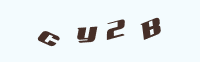# 《侠客风云传前传》队友、武功、道具及武器代码

《侠客风云传前传》刷心法内功及道具等很麻烦？今天小编要为大家带来的是玩家“芙兰茗璇“分享的《侠客风云传前传》队友、武功、道具及武器代码，一起来看看吧!正常数据的队友，正常游戏可入队的角色。

100008=凌香儿

100017=无瑕子

100019=老胡

100026=醉仙

100027=橘叟

100028=神医

100029=仙音

100030=书生

100031=丹青

100033=沈澜

100037=方云华

100054=任清璇

100056=纪玟

100058=蓝婷

100059=史燕

100061=风吹雪

100065=史刚

100066=史义

100072=西门峰

100075=江瑜

100078=关伟

100081=唐冠南

[为游戏媒体贡献点力量呗]

## 评论(0条评论)